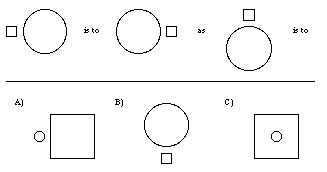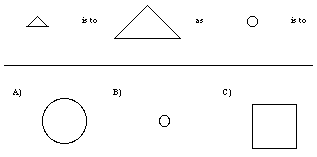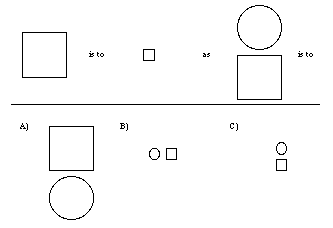## CSC 550/650: Assignment 2

### Relations & Prolog Programs

This assignment involves writing and reasoning with numerous Prolog programs. As part of the documentation for your code, you should include a short English description of each relation that you define. These descriptions should concentrate on the declarative content of each relation (i.e. on what is being defined), rather than on the procedural aspects (i.e. on how it "works").

Your aim in solving these problems should be first to produce the clearest possible solution and only second to make the solution efficient. Solutions for each problem must be clearly separated out. Programs that are difficult to read or understand will be penalized.

1. Consider the following Prolog program, which defines relations between cities (similar to the example discussed in class). Trace by hand the sequence of resolution steps involved in solving the query ?- connected(X,X). That is, show the list of goals at each step in the Prolog resolution, noting any bindings that occur and also any backtracking that takes place. See the lecture notes for similar examples tracing queries about family relations. road(a, b). road(b, c). road(b, a). road(c, d). connected(City1, City2) :- road(City1, City2). connected(City1, City2) :- road(City1, City3), connected(City3, City2).
2. Write Prolog facts and/or rules to define the relation sum_range(Low, High, Sum), where Sum is the sum of all the integers between Low and High, inclusive. If Low > High, the Sum is assumed to be 0. For example: ?- sum_range(1, 100, Sum). ?- sum_range(5, 3, Sum). Sum = 5050 Sum = 0 Yes Yes

Hint: to compute the sum from Low to High, recursively compute the sum from Low+1 to High and then add Low. Be sure that your relation does not produce incorrect answers on backtracking (e.g., if the user hits a semi-colon).

3. As suggested by several examples from the lectures, one possible use of Prolog is in providing an intelligent front-end to a database. Assume that you are given a set of Prolog facts of the following form, describing courses offered at Creighton. course(Name, loc(Bldg,Room), time(Day,StartHour:StartMin,EndHour:EndMin), instr(FirsName,LastName)). where : is a standard Prolog operator. For instance, the courses taught by Dr. Reed in Spring 2002 could be described using the facts: course(csc107,loc(old_gym,411),time(tue,14:00,15:15),instr(dave,reed)). course(csc107,loc(old_gym,411),time(thu,14:00,15:15),instr(dave,reed)). course(csc551,loc(old_gym,411),time(mon,15:30,16:45),instr(dave,reed)). course(csc551,loc(old_gym,411),time(wed,15:30,16:45),instr(dave,reed)). course(csc550,loc(old_gym,411),time(tue,17:00,19:45),instr(dave,reed)). course(csc650,loc(old_gym,411),time(tue,17:00,19:45),instr(dave,reed)). Your task is to build a simple interface to this database by writing Prolog facts and/or rules to define the following relations:

• is_instructor(LastName,Course): which is true if Course is taught by a person whose last name is LastName. ?- is_instructor(Instr,csc550). ?- is_instructor(reed,Class). Instr = reed Class = csc107 ; Yes Class = csc107 ; Class = csc551 Yes
• is_teaching(LastName,Period): which is true if LastName teaches a class at the specified Period. ?- is_teaching(reed,Period). ?- is_teaching(Who,time(tue,17:00,19:45)). Period = time(mon,15:30,16:45) ; Who = reed Period = time(wed,15:30,16:45) ; yes Period = time(tue,14:00,15:15) Yes
• is_busy(LastName,Day,H:M,Location): which is true if LastName is busy at Location on Day at the time H:M. ?- is_busy(reed,wed,15:00,Where). ?- is_busy(Instr,tue,17:30,loc(old_gym,411)). Where = loc(old_gym,411) Instr = reed yes yes Note: you may assume that the day and time will both be fully instantiated in queries to is_busy, i.e. contain no variables. You may also assume that all classes end on the same day they begin (i.e., no classes extend beyond midnight).

4. A (labeled) binary tree is a recursive structure that is either (1) empty, or else (2) consists of a labeled root, a left subtree, and a right subtree. Similar to lists, trees are amenable to representation in the predicate calculus. For example, we may represent an empty tree by the constant void, and a non-empty tree by the term tree(Root,Left,Right) where Root is the label of the root and Left and Right are representations of the left and right subtrees, respectively. Thus the tree with root a and leaves b and c would be represented by the term tree(a,tree(b,void,void),tree(c,void,void)).

• Write Prolog facts and/or rules to define the relation is_tree(T), which is true if T is a term representing a valid tree. ?- is_tree(tree(a,void,void)). ?- is_tree(tree(a,b,void)). Yes No
• Write Prolog facts and/or rules to define the relation contains(T,X), which is true if tree T contains the value X at any node. Hint: a non-empty tree contains a particular value if it is stored at the root or if one of its subtrees contains the value. ?- contains(tree(a,void,void),a). ?- contains(tree(a,void,void),b). Yes No
• Write Prolog facts and/or rules to define the relation num_nodes(T,N), which is true if tree T contains N nodes. Hint: the number of nodes in a non-empty tree is the sum of the number of nodes in the two subtrees plus 1 (for the root node). ?- num_nodes(tree(a,void,void),N). ?- num_nodes(T,0). N = 1 T = void Yes Yes

5. Consider the IQ test analogy reasoner described in class, which can be copied from online. You are to modify/extend this program in the following ways:

• First, refine the transformations so that a unique answer is obtained for question 4. As you may recall, the source of the current ambiguity is that the transformations invertPosition and invertSizes produce the same result when applied to figures of the same shape (e.g., a small square above a large square). One way to resolve this would be to limit one of the transformations, say invertSizes, to instances where the two figures are different shapes. This would involve adding a premise to the transformation, i.e., converting the fact to a rule with a premiss that assumes different shapes.

• Add a relation so that figures can be described as being to the left of one another. Note: this will require updating the transformations to make use of this new relation.

• Add further transformations that will enable the program to solve the questions that appear below (whose "correct" answers are B, A, and C, respectively).

You are certainly free (and encouraged) to add more to this program to make it even more powerful/interesting.